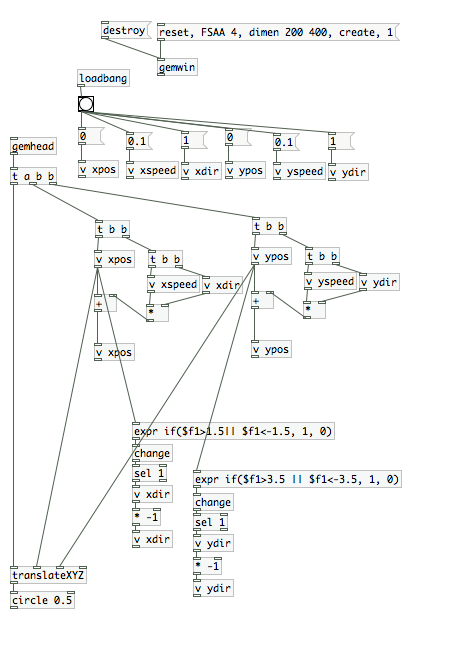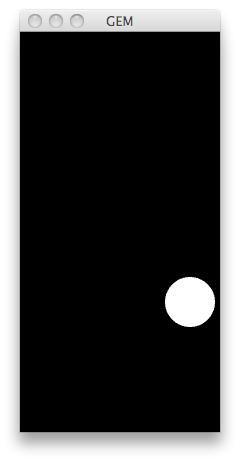## 2011年3月28日 星期一

### processing的基本繪圖_9 bounce

int size = 60;       // Width of the shape
float xpos, ypos;    // Starting position of shape

float xspeed = 2.8;  // Speed of the shape
float yspeed = 2.2;  // Speed of the shape

int xdirection = 1;  // Left or Right
int ydirection = 1;  // Top to Bottom

void setup()
{
size(640, 200);
noStroke();
frameRate(30);
smooth();
// Set the starting position of the shape
xpos = width/2;
ypos = height/2;
}

void draw()
{
background(102);

// Update the position of the shape
xpos = xpos + ( xspeed * xdirection );
ypos = ypos + ( yspeed * ydirection );

// Test to see if the shape exceeds the boundaries of the screen
// If it does, reverse its direction by multiplying by -1
if (xpos > width-size || xpos < 0) {
xdirection *= -1;
}
if (ypos > height-size || ypos < 0) {
ydirection *= -1;
}

// Draw the shape
ellipse(xpos+size/2, ypos+size/2, size, size);
}